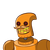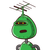# A farmer borrowed Rs. 25,000 from a sahakari bank at 3 p.c.p.a. What amount he will pay to the bank at the end of the year? * October 17, 2021 by Remi

A farmer borrowed Rs. 25,000 from a sahakari bank at 3 p.c.p.a. What amount he will pay to the bank at the end of the year? *
2 points
Rs. 27570
Rs. 25750
Rs. 25570
Rs. 27750​

### 2 thoughts on “A farmer borrowed Rs. 25,000 from a sahakari bank at 3 p.c.p.a. What amount he will pay to the bank at the end of the year? *<br /”

1.## Solution:-

━━━━━━━━━━━━━━━━━━━━━━━━━━

$$\large\to{\underbrace{\underline{\sf{Understanding\:the\:concept:-}}}}$$

◍ Here, the question has given us the principal, rate of interest and time that is Rs. 25,000, 3% per annum and for 1 year respectively. Now, the question has asked us to find the amount he will pay back. So to get the answer we need to apply the formula of simple interest first. And after it follow the steps given below.

◈ The Simple Interest is Rs. 750.

◈ The amount he will pay back is Rs. 25,750.

### GIVEN:-

☆ Principal = Rs. 25,000

☆ Rate of Interest = 3% per annum

☆ Time = 1 year

### TO FIND:-

↠Amount he will pay back = ?

### FORMULA:-

⬤ Simple Interest = P × R × T / 100

⬤ Amount he will pay back = SI + Principal

### SOLVING BY APPLYING THE FORMULA:-

⇨ Principal = Rs. 25,000

⇨ Rate = 3%

⇨ Time = 1 year

⬤ Simple Interest = P × R × T / 100

• Finding the SI:

➢ Simple Interest = 25,000 × 3 × 1 / 100

➢ Simple Interest = 25,000 × 3 / 100

➢ Cancelling the zeros.

➢ Simple Interest = 250 × 3 / 1

➢ Simple Interest = 250 × 3

➢ Simple Interest = 250 × 3 = 750

➢ Simple Interest = Rs. 750

Thus, the SI is Rs. 750.

⇨ Amount = Principal + Simple Interest

➢ Amount = 25,000 + 750

➢ Amount = 25,000 + 750 = 25,750

➢ Amount = Rs. 25,750

Hence, we got the answer. The Amount is Rs. 25,750.

━━━━━━━━━━━━━━━━━━━━━━━━━━

2.Step-by-step explanation:

P= 25000

r= 3%

t= 1 year

SI= r×t/100= 25000× 3×1/100= 250×3= 750

Amount= SI + P

= 750+25000

= 25750